## Matrices

Much of the machinery of linear algebra involves matrices, which are rectangular arrays of numbers.

A rectangular array of numbers, enclosed in a large pair of either parentheses or brackets, such asis called a matrix. The size or dimensions of a matrix are specified by stating the number of rows and the number of columns it contains. If the matrix consists of m rows and n columns, it is said to be an m by n (written m x n) matrix. For example, the matrices above are 2 by 3, since they contain 2 rows and 3 columns:Note that the rows are counted from top to bottom, and the columns are counted from left to right.

The numbers in the array are called the entries of the matrix, and the location of a particular entry is specified by giving first the row and then the colun where it resides. The entry in row i, column j is called the (i, j) entry. For example, since the entry −2 in the matrix above is in row 2, column 1, it is the (2, 1) entry. The (1, 2) entry is 0, the (2, 3) entry is 1, and so forth. In general, the (i, j) entry of a matrix A is written a ij , and the statement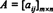indicates that A is the m x n matrix whose (i, j) entry is a ij .

Example 1: The set of all m x n matrices whose entries are real numbers is denoted M m x n ( R). If A ε M 2x3 ( R), how many entries does the matrix A contain?

Since every matrix in M 2x3(R) consists of 2 rows and 3 columns, A will contain 2 x 3 = 6 entries. An example of such a matrix isExample 2: If B is the 2 x 2 matrix whose (i, j) entry is given by the formula b ij = (−1) i+j ( i+j), explicitly determine B.

The (1, 1) entry of B is b 11 = (−1) 1+1 (1+1) = 2; the (1, 2) entry is b = (−1) 1+2(1+2) = −3; the (2, 1) entry is also −3; and the (2, 2) entry is b 22 = (−1) 22(2+2) = 4. Therefore,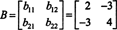Example 3: Give the 3 x 3 matrix whose (i, j) entry is expressed by the formula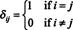ij is called the Kronecker delta.)

The (1,1), (2,2), and (3,3) entries are each equal to 1, but all other entries are 0. Thus, the matrix is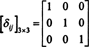Entries along the diagonal. Any entry whose column number matches its row number is called a diagonal entry; all other entries are called off‐diagonal. The diagonal entries in each of the following matrices are highlighted: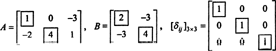In the matrix A, the diagonal entries are a 11 = 2 and b 22 = 4; in B, the diagonal entries are b 11 = 2 and b 22 = 4; and in the matrix [δ ij ] 3x3, the diagonal entries are δ 11 = δ 22 = δ 33 = 1.

If every off‐diagonal entry of a matrix equals zero, then the matrix is called a diagonal matrix. For example, the matrix [δ ij ] 3x3 above is a diagonal matrix. It is not uncommon in such cases, particularly with large matrices, to simply leave blank any entry that equals zero. For example,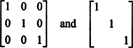are two ways of writing exactly the same matrix. Blocks of zeros are often left blank in nondiagonal matrices also. An n x n diagonal matrix whose entries—from the upper‐left to the lower‐right—are a 11, a 22,…, a nn is often written Diag(a 11, a 22,…, a nn ).

Square matrices. Any matrix which has as many columns as rows is called a square matrix. The 2 x 2 matrix in Example 2 and the 3 x 3 matrix in Example 3 are square. If a square matrix has n rows and n columns, that is, if its size is n x n, then the matrix is said to be of order n .

Triangular matrices. If all the entries below the diagonal of a square matrix are zero, then the matrix is said to be upper triangular. The following matrix, U, is an example of an upper triangular matrix of order 3:If all the entries above the diagonal of a square matrix are zero, then the matrix is said to be lower triangular. The following matrix, L, is an example of a lower triangular matrix of order 4: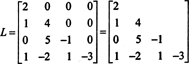A matrix is called triangular if it is either upper triangular or lower triangular. A diagonal matrix is one that is both upper and lower triangular.

The transpose of a matrix. One of the most basic operations that can be performed on a matrix is to form its transpose. Let A be a matrix; then the transpose of A, a matrix denoted by A T, is obtained by writing the rows of A as columns. More precisely, row i of A is column i of A T (which implies that column j of A is row j of A T). If A is m x n, then A T will be n x m. Also, it follows immediately from the definition that ( A T) T = A.

Example 4: The transpose of the 2 x 3 matrixis the 3 x 2 matrixExample 5: Note that each of the matrices in Examples 2 and 3 is equal to its own transpose: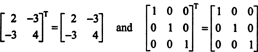Any matrix which equals its own transpose is called a symmetric matrix.

Row and column matrices. A matrix that consists of precisely one row is called a row matrix, and a matrix that consists of precisely one column is called a column matrix. For example,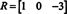is a row matrix, while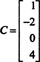is a column matrix. Note that the transpose of a row matrix is a column matrix and vice versa; for example,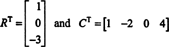Row and column matrices provide alternate notations for a vector. For example, the vector v = (2,−1,6) in R 3 can be expresed as either a row matrix or a column matrix: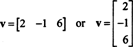It is common to denote such a matrix by a bold, lower‐case (rather than an italic, upper‐case) letter and to refere to it as either a row vector or a column vector.

Zero matrices. Any matrix all of whose entries are zero is called a zero matrix and is generically denoted O. If it is improtant to explicitly indicate the size of a zero matrix, then subscript notation is used. For example, the 2 x 3 zero matrixwould be written O 2x3. If a zero matrix is a row or column matrix, it is usually denoted O, which is consistent with the designation of O as the zero vector.